Next: 3.3.5 Thermoelectric Figure of Merit Up: 3.3 Thermoelectrics Engineering in ZGNRs Previous: 3.3.3 The Role of Line-Edge-Roughness   Contents

## 3.3.4 The Effect of Roughness on Phonon Transport

The high thermal conductivity of graphene is mostly due to the lattice contribution, whereas the electronic contribution is much smaller [29,28]. In order to reduce the thermal conductivity, therefore, the focus is placed on reducing phonon conduction. Although the reduction in the electronic transmission of channels with ELDs can be quite strong when considering edge roughness, the reduction in the lattice part of the thermal conductivity is even stronger. One can take advantage of this effect when attempting to optimize the thermoelectric figure of merit.

For the phonon modes, the dynamic matrix is constructed using the fourth nearest-neighbor force constant model . The force constant method uses a set of empirical fitting parameters and can be easily calibrated to experimental data. The fitting parameters given in Ref.  are used for graphene-based structures. Here, it is assumed that this model is still valid for structures that include ELDs. Although verification of its validity for ELD-ZGNRs has not been demonstrated yet, for instance using first-principle calculations, in Ref.  it was shown using DFT simulations that there is little difference between the phonon transmission of carbon nanotube structures with and without ELDs which could justify the model employed here. In any case, as shown below, the main influence on the phonon transport in this work originates from edge roughness scattering, which reduces the phonon transmission drastically. The effect of edge roughness scattering can be captured adequately by the model employed in this work. The influence of the ELDs on the phonon transmission is much smaller than the effect of edge roughness, and therefore usage of the numerically less expensive fourth nearest-neighbor force constant method is justified.

The phonon transmission for the edge roughened ELD-ZGNR(10,10) channel versus energy is shown in Fig. 3.25-a. Results for channel lengths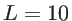,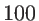, and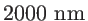are shown. As expected, the transmission decreases as the length is increased. What is important, however, is that the decrease is much stronger than the decrease of the electron transmission shown in Fig. 3.23-a. For example, for a channel length of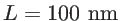the phonon transmission reduces by more than a factor of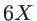, whereas the electronic transmission even at larger length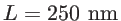reduces only by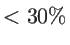. Interestingly, the same order of reduction of the phonon transmission is observed for the 2ELD-ZGNRs as shown in Fig. 3.25-b, indicating that the line defect does not affect phonon conduction significantly compared to the effect of edge roughness.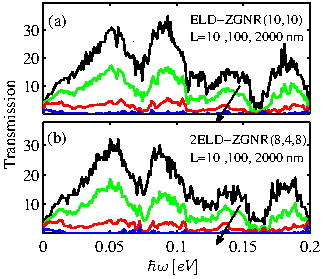The denominator of the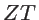figure of merit consists of the sum of the contributions to the thermal conductivity of the electronic system and the phononic system. In graphene the phonon part dominates the thermal conductivity, whereas the electronic part contribution is much smaller. The situation is different, however, in rough ELD-ZGNRs, in which the phonon thermal conductivity is degraded more than the electronic thermal conductivity. Figure 3.26 clearly illustrates this effect by showing the ratio of the phonon thermal conductance to the electronic thermal conductance versus the rough channel length. The cases of ELD-ZGNR(10,10) and 2ELD-ZGNR(8,4,8) are shown in dashed-red and dash-dot-blue lines, respectively. For small channel lengths, where transport is quasi-ballistic and roughness does not affect the transmission significantly,is almost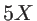larger than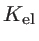. As the length of the channel increases and the effect of the roughness becomes significant, the phonon system is degraded more than the electronic system, and theis significantly reduced compared to. For lengths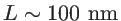and beyond,can become even smaller than. The trend is the same when considering channels with one or two ELDs.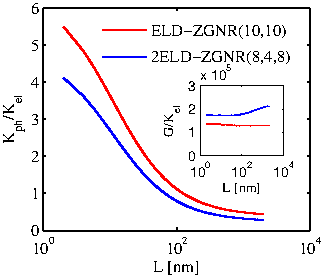The inset of Fig. 3.26 shows that the ratio of the electrical conductance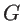overis almost constant, from which it can be indicated that bothandfollow the same trend, as dictated by the Wiedemann-Franz law. Theandvalues used in Fig. 3.25 are extracted using the corresponding mean free paths (MFPs) for phonons and electrons respectively, defined as Eq. 3.2. Alternatively,andcould be extracted from the transmission calculations by using a statistical average over several rough samples for each channel length. The results of both methodologies are in good agreement for the electronic part of the thermal conductivity. For the lattice part, the agreement is good only for the shorter channels, below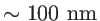. For larger channel lengths, the phonon transmission is severely reduced which increases the relative statistical error in the calculation for extracting the. The values extracted directly from the integration of the average phonon transmission might be as much aslarger. In this case the ratio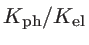will be closer to unity, but this is still a huge advantage compared to devices without roughness.Next: 3.3.5 Thermoelectric Figure of Merit Up: 3.3 Thermoelectrics Engineering in ZGNRs Previous: 3.3.3 The Role of Line-Edge-Roughness   Contents
H. Karamitaheri: Thermal and Thermoelectric Properties of Nanostructures